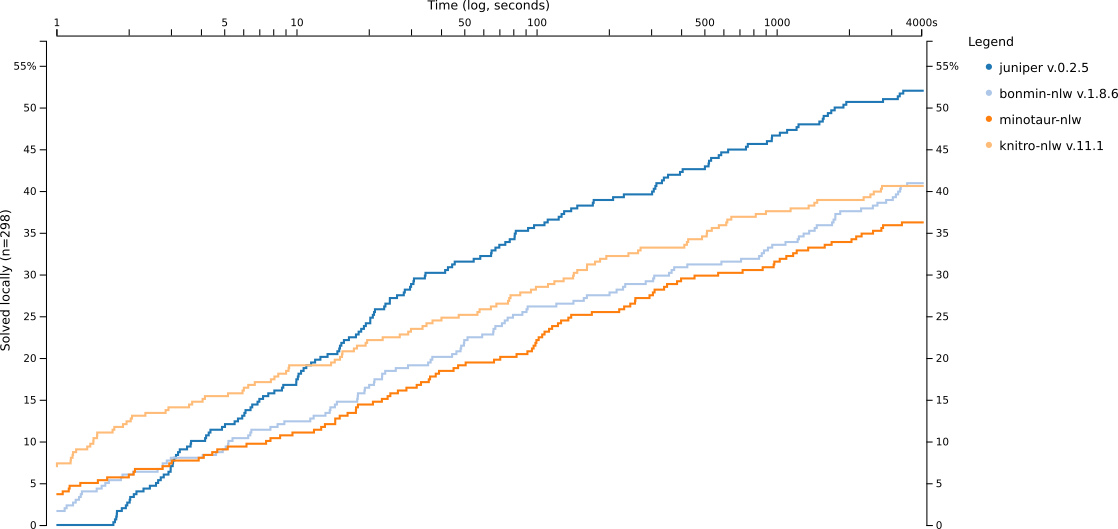# Juniper

Juniper (Jump Non linear Integer Program solver) is a solver for MixedIntegerNonLinearPrograms (MINLPs) written in Julia. Juniper solves these kind of problems using a NLP solver and then branch and bound. If the NLP solver isn't global optimal then Juniper is a heuristic. You need the global optimum? Check out Alpine.jl

# Why?

You have a non linear problem with discrete variables (MINLP) and want some more control over the branch and bound part? => Use Juniper

You have a really good solver for the relaxation and just want to solve problems with discrete variables as well? Just combine your solver with Juniper.

# Basic usage

] add Juniper as ] is used to get to interact with the package manager.

using Juniper

You also have to import your NLP solver i.e.

using Ipopt

as well as JuMP

Define Juniper as the optimizer:

optimizer = Juniper.Optimizer
nl_solver = optimizer_with_attributes(Ipopt.Optimizer, "print_level" => 0)

And give it a go:

using LinearAlgebra # for the dot product
m = Model(optimizer_with_attributes(optimizer, "nl_solver"=>nl_solver))

v = [10,20,12,23,42]
w = [12,45,12,22,21]
@variable(m, x[1:5], Bin)

@objective(m, Max, dot(v,x))

@NLconstraint(m, sum(w[i]*x[i]^2 for i=1:5) <= 45)

optimize!(m)

# retrieve the objective value, corresponding x values and the status
println(JuMP.value.(x))
println(JuMP.objective_value(m))
println(JuMP.termination_status(m))

This solver is a NLP solver therefore you should have at least one NLconstraint or NLobjective.

Juniper is specialized for non convex problems which get solved locally optimal. It will solve convex problems as well but specialized solvers for convex problems should be preferred then.

# Performance

You can find detailed performance measurements in our paper or technical report.

The most recent stats can be found on the website BnBVisual.